Fig. represents vertical sections of four wings moving horizontally in air. In which case the force is upwards

(a)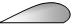(b)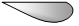(c)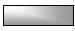(d)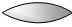Concept Questions :-

Bernoulli theorem
High Yielding Test Series + Question Bank - NEET 2020

Difficulty Level:

A tank is filled with water up to a height H. Water is allowed to come out of a hole P in one of the walls at a depth D below the surface of water. Express the horizontal distance x in terms of H and D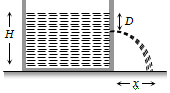(a) $\mathrm{x}=\sqrt{\mathrm{D}\left(\mathrm{H}-\mathrm{D}\right)}$

(b) $\mathrm{x}=\sqrt{\frac{\mathrm{D}\left(\mathrm{H}-\mathrm{D}\right)}{2}}$

(c) $\mathrm{x}=2\sqrt{\mathrm{D}\left(\mathrm{H}-\mathrm{D}\right)}$

(d) $\mathrm{x}=4\sqrt{\mathrm{D}\left(\mathrm{H}-\mathrm{D}\right)}$

Concept Questions :-

Bernoulli theorem
High Yielding Test Series + Question Bank - NEET 2020

Difficulty Level:

A rectangular vessel when full of water takes 10 minutes to be emptied through an orifice in its bottom. How much time will it take to be emptied when half filled with water
(a) 9 minute                        (b) 7 minute
(c) 5 minute                        (d) 3 minute

Concept Questions :-

Bernoulli theorem
High Yielding Test Series + Question Bank - NEET 2020

Difficulty Level:

A streamlined body falls through air from a height h on the surface of a liquid. If d and D(D > d) represents the densities of the material of the body and liquid respectively, then the time after which the body will be instantaneously at rest, is

(a) $\sqrt{\frac{2\mathrm{h}}{\mathrm{g}}}$                          (b) $\sqrt{\frac{2\mathrm{h}}{\mathrm{g}}·\frac{D}{d}}$

(c) $\sqrt{\frac{2\mathrm{h}}{\mathrm{g}}·\frac{d}{D}}$                    (d) $\sqrt{\frac{2\mathrm{h}}{\mathrm{g}}}\left(\frac{d}{D-d}\right)$

Concept Questions :-

Archimedes principle
High Yielding Test Series + Question Bank - NEET 2020

Difficulty Level:

A large tank of cross-section area A is filled with water to a height H. A small hole of area 'a' is made at the base of the tank. It takes time ${\mathrm{T}}_{1}$ to decrease the height of water to $\frac{\mathrm{H}}{\mathrm{\eta }}\left(\mathrm{\eta }>1\right)$ ; and it takes ${\mathrm{T}}_{2}$ time to take out the rest of water. If ${\mathrm{T}}_{1}={\mathrm{T}}_{2}$, then the value of $\mathrm{\eta }$ is
(a) 2                                    (b) 3
(c) 4                                    (d) $2\sqrt{2}$

Concept Questions :-

Bernoulli theorem
High Yielding Test Series + Question Bank - NEET 2020

Difficulty Level:

As the temperature of water increases, its viscosity

(a) Remains unchanged

(b) Decreases

(c) Increases

(d) Increases or decreases depending on the external pressure

Concept Questions :-

Viscosity
High Yielding Test Series + Question Bank - NEET 2020

Difficulty Level:

A small drop of water falls from rest through a large height h in air; the final velocity is

(a) $\propto \sqrt{\mathrm{h}}$

(b) $\propto \mathrm{h}$

(c) $\propto \left(1/\mathrm{h}\right)$

(d) Almost independent of h

Concept Questions :-

Viscosity
High Yielding Test Series + Question Bank - NEET 2020

Difficulty Level:

The rate of flow of liquid in a tube of radius r, length l, whose ends are maintained at a pressure difference P is $\mathrm{V}=\frac{{\mathrm{\pi QPr}}^{4}}{\mathrm{\eta l}}$ where $\mathrm{\eta }$ is coefficient of the viscosity and Q is

(a) 8                      (b) $\frac{1}{8}$

(c) 16                     (d) $\frac{1}{16}$

Concept Questions :-

Viscosity
High Yielding Test Series + Question Bank - NEET 2020

Difficulty Level:

Water flows in a streamlined manner through a capillary tube of radius a, the pressure difference being P and the rate of flow Q. If the radius is reduced to a/2 and the pressure increased to 2P, the rate of flow becomes

(a) $4\mathrm{Q}$                          (b) $\mathrm{Q}$

(c) $\frac{\mathrm{Q}}{4}$                          (d) $\frac{\mathrm{Q}}{8}$

Concept Questions :-

Viscosity
High Yielding Test Series + Question Bank - NEET 2020

Difficulty Level:

Water is flowing in a pipe of diameter 4 cm with a velocity 3 m/s. The water then enters into a tube of diameter 2 cm. The velocity of water in the other pipe is
(a) 3 m/s                                        (b) 6 m/s
(c) 12 m/s                                       (d) 8 m/s

Concept Questions :-

Equation of continuity